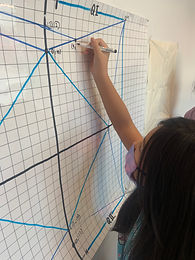## Mr. Rodney

### Target 1​

###### Lesson Type:

New

Geometry

:

Symmetry

Create symmetrical figures (using understandings that a figure does not change in a symmetrical transformation).

###### 1:

Define reflectional symmetry.

###### 2:

Define rotational symmetry.

###### 3:

Understand that an object has rotational symmetry if there is a center point around which the object is turned less than 360 degrees and the object looks the same.

###### 4:

Define reflectional symmetry.

4th

###### Vocabulary:

Reflectional Symmetry, Rotational Symmetry

Activities:

• Discussed and defined "symmetry" as well as the differences between rotaional and reflectional symmetry.
• As a class, took turns giving clear and descriptive instructions for each step of folding a "fortune teller," practicing turn taking and communication and listening skills.
• Unfolded the origami paper to study the lines and creases to determine if they were examples of reflectional or rotational symmetry.
• Incorporated fractions and its vocabulary by answering question such as ":Each triangle in the fortune teller represecents what fraction of the whole paper?"### Home Exploration

###### Guiding Questions:## Absent Students:

### Target 2

:

###### 1:

Identify the x- and y-axis on a coordinate plane.

###### 2:

Locate points in all four quadrants of the coordinate plane.

###### 3:

Understand that the first number in a coordinate pair indicates placement on the x-axis and the second indicates placement on the y-axis.

###### 4:

Graph points in the first and second quadrant of the coordinate plane.

5th

###### Vocabulary:

Coordinates, X- and Y-Axis, Ordered Pair

Activities:

• In tandem with the lesson on Symmetry, students were tasked with the challenge of recreating the creases on an origami paper onto the coordinate plane.
• Students studied the folds of an unfolded paper crane and related the first two folds to what would be the x- and y-axis.
• Took turns identifying intersection creases to determine the next logical points that could be plotted.
• Properly labeled each point drawn with its ordered pair.### Home Exploration

###### Guiding Questions:### Target 3

:

###### Vocabulary:

Activities:### Home Exploration